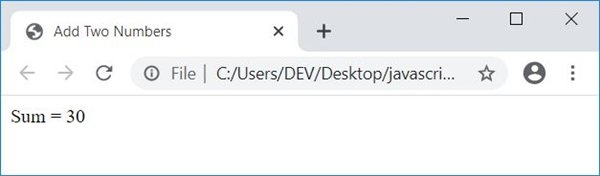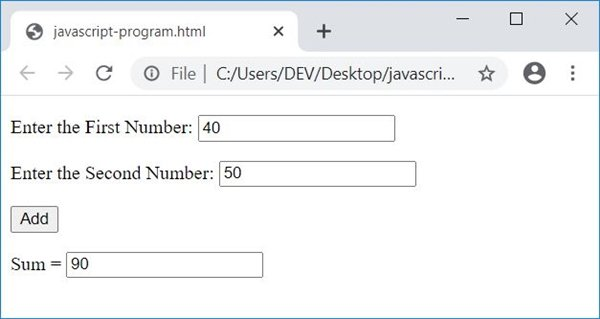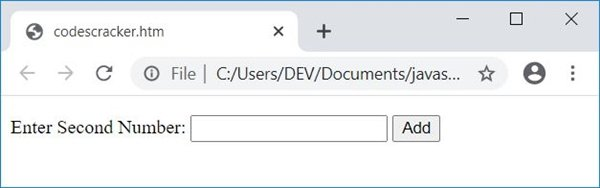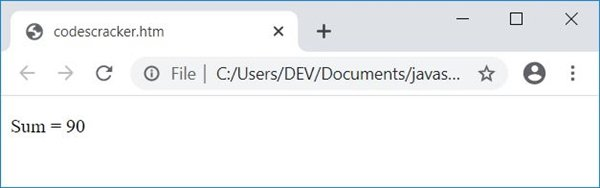In this article, you will learn and get code on addition of two numbers in JavaScript. The program is created in following ways:

• Simple JavaScript Code to Add two Numbers
• Add Two Numbers in a WebPage
• Add Two Numbers using Form and TexBox
• Receive input from user one by one

## Add Two Numbers in JavaScript

Here is the simplest JavaScript code to add two numbers.

```var numOne = 10;
var numTwo = 20;
var sum = numOne+numTwo;```

As you can see from the above three lines of JavaScript code. The value 10 gets initialized to numOne. So numOne=10. And the value 20 gets initialized to numTwo. So numTwo=20. Now the statement, numOne+numTwo or 10+20 or 30 gets initialized to sum. So sum=30, which is the addition of two numbers stored in numOne and numTwo

On same concept as mentioned above, I'm going to create another JavaScript code that also adds two numbers and displays the result as an HTML output.

## JavaScript Add Two Numbers in HTML

Here is the JavaScript program that adds two numbers and displays the addition result in a webpage using HTML:

```<!doctype html>
<html>
<script>
var numOne = 10;
var numTwo = 20;
var sum = numOne+numTwo;
document.write("Sum = " + sum);
</script>
<body>

</body>
</html>```

Save this code in a file with .html extension. Open the file in a web browser. Here is the output you will see:Note - The document.write() method writes the value passed in its braces (), into an HTML output.

## JavaScript Add Two Numbers using Form and TextBox

This is the actual program to add two numbers in JavaScript using form and TextBoxes. As this program allows user to input the data. On the basis of user input, JavaScript code gets in action to output the addition result

```<!doctype html>
<html>
<script>
{
var numOne, numTwo, sum;
numOne = parseInt(document.getElementById("first").value);
numTwo = parseInt(document.getElementById("second").value);
sum = numOne + numTwo;
}
</script>
<body>

<p>Enter the First Number: <input id="first"></p>
<p>Enter the Second Number: <input id="second"></p>

</body>
</html>```

Here is its sample run with user input, 40 as first number and 50 as second number:When user clicks on the button, Add, then a function named add() gets called. And all the statements of this function gets executed. The following statement:

`numOne = parseInt(document.getElementById("first").value);`

states that, an int (integer) value of an HTML element whose id is first gets initialized to numOne. And the statement:

`document.getElementById("answer").value = sum;`

states that, the value of sum gets printed to an HTML element with id, answer.

#### Live Output of Previous Program

And here is the live output of above JavaScript code on addition of two numbers:

Enter the First Number:

Enter the Second Number:

Sum =

### Get User Input one by one

Let's create one more interesting JavaScript program that does the same job as of previous program, that is receives two numbers from user, and output its addition result.

This program receives input from user in a way that, user is allowed to input one at a time.

```<!doctype html>
<html>
<script>
var numOne, numTwo, sum;
function getFirNum()
{
numOne = parseInt(document.getElementById("first").value);
if(numOne)
{
temp = document.getElementById("paraOne");
temp.style.display = "none";
temp = document.getElementById("paraTwo");
temp.style.display = "block";
}
}
function getSecNum()
{
numTwo = parseInt(document.getElementById("second").value);
if(numOne && numTwo)
{
temp = document.getElementById("paraOne");
temp.style.display = "none";
temp = document.getElementById("paraTwo");
temp.style.display = "none";
sum = numOne + numTwo;
temp = document.getElementById("paraThree");
temp.style.display = "block";
document.getElementById("res").innerHTML = sum;
}
}
</script>
<body>

<p id="paraOne">Enter First Number: <input id="first">
<button onclick="getFirNum()">Enter</button></p>
<p id="paraTwo" style="display:none;">Enter Second Number: <input id="second">
<p id="paraThree" style="display:none;">Sum = <span id="res"></span></p>

</body>
</html>```

Here is the snapshot that shows initial output produced by above JavaScript program on addition of two numbers entered by user:Now enter the first number say 50 and click on Enter button. Here is the output after performing this:Now enter the second number say 40 and click on Add button. Here is the output that shows the addition result of entered two numbers:The following code:

`style="display:none;"`

is a CSS code, that hides an HTML element where it is included. Because it is included in a p (paragraph) tag whose id is paraTwo, so this paragraph gets hidden initially. The following JavaScript code:

`temp.style.display = "block";`

states that, an HTML element whose id is stored in temp variable, gets visible after executing this statement. And the following statement:

`temp.style.display = "none";`

works same as previous. The only difference is, this statement hides an HTML element. And the JavaScript code:

`document.getElementById("res").innerHTML = sum;`

states that, the content of an HTML element with id res gets replaced with the value of sum.